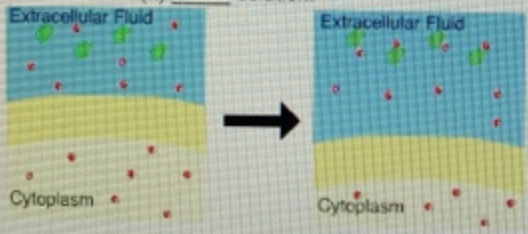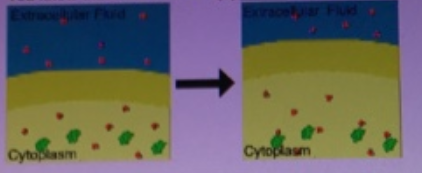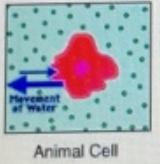# Problem: Part A What name is given to the process by which water crosses a selectively permeable membrane? a) diffusion b) pinocytosisc) phagocytosisd) passive transport e) osmosis  Part B This cell is in a(n) ________ solution. a) hypotonicb) isotonicc) hypertonic d) hypertonic or isotonic e) hypotonic and isotonic Part C You know that this cell is in a(n)_______ solution because the cell ______. a) hypertonic .... lost water b) hypertonic .... gained waterc) hypotonic .... swelledd) hypotonic .... shrunke) isotonic .... neither lost nor gained wtaerPart DYou know that this cell is in a(n) ______ solution because it _______. a) hypotonic .... lysedb) hypertonic .... gained waterc) hypertonic .... lysedd) hypertonic solution .... lost watere) hypotonic ... is turgid

###### FREE Expert Solution

Part A: Osmosis – movement of solvent molecules across a selectively-permeable membrane

– solvent molecules move from lower to higher solute concentration

Answer: e) Osmosis is the movement of water across a selectively permeable membrane.###### Problem Details

Part A

What name is given to the process by which water crosses a selectively permeable membrane?

a) diffusion

b) pinocytosis

c) phagocytosis

d) passive transport

e) osmosis

Part B

This cell is in a(n) ________ solution.a) hypotonic

b) isotonic

c) hypertonic

d) hypertonic or isotonic

e) hypotonic and isotonic

Part C

You know that this cell is in a(n)_______ solution because the cell ______.a) hypertonic .... lost water

b) hypertonic .... gained water

c) hypotonic .... swelled

d) hypotonic .... shrunk

e) isotonic .... neither lost nor gained wtaer

Part D

You know that this cell is in a(n) ______ solution because it _______.a) hypotonic .... lysed

b) hypertonic .... gained water

c) hypertonic .... lysed

d) hypertonic solution .... lost water

e) hypotonic ... is turgid